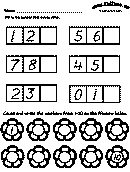## lbartman.com - the pro math teacher

• Subtraction
• Multiplication
• Division
• Decimal
• Time
• Line Number
• Fractions
• Math Word Problem
• Kindergarten
• a + b + c

a - b - c

a x b x c

a : b : c

# Free Kindergarten Math Worksheets

Public on 11 Oct, 2016 by Cyun Lee

###free printable kindergarten math worksheets

Name : __________________

Seat Num. : __________________

Date : __________________

### HOW MANY STARS EACH LINE ?

......
......
......
......
......
show printable version !!!hide the show

## RELATED POST

Not Available

## POPULAR

free printable subtraction worksheets for kindergarten

math linear equations worksheets

problem solving addition and subtraction worksheets

subtracting time worksheets

multiplication worksheets ks3